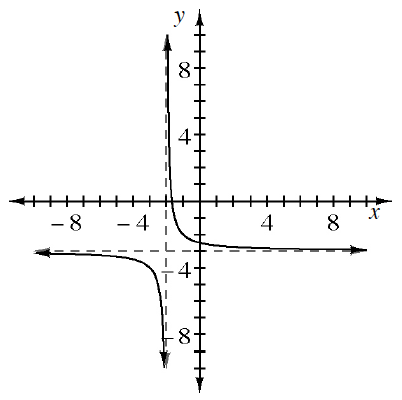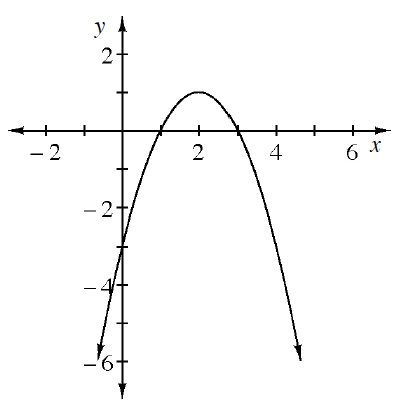### Home > PC3 > Chapter Ch8 > Lesson 8.1.3 > Problem8-52

8-52.

Write an equation for each curve below. State the equation of the parent function and describe the transformation.

1.$y=\frac{a}{x-h} +k$

1.$y = a(x-h)^2+k$